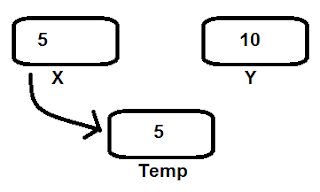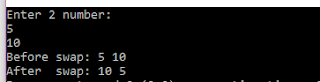# How to swap 2 number ?

There is two number in two variable. We have to swap the position of the numbers. For example, theSwap
variable x holding integer value 5 and another variable y holding the integer value 10. Now we have to swap the position of those two numbers.

#### Logic:

We can use another temporary variable to store the value of x.Swap fig: 01
Now we have a backup copy of the value of x into temp variable.
So we can now give the value of y to x.Swap fig: 02
Yes, we have done half of our job!!  x is now holding the value of y. Now time to assign the value of x into y. Remember, we have save the value of x into temp variable at figure 01. So now put the temp value to y.Swap fig: 03
Oh yes. Now look..!!! We have successfully swap the value of x to y and value of y to x.

### Now it's time to check what we understand. Write a program to swap two number:

[Note: Don't see the code first. Please try yourself ]

#### Sample Input:Sample Input
The program should take input from user.

#### Sample Output:Sample Output

Don't worry. If you failed, the code is here. Hope you will understand the code easily.

-----Happy Coding-----

How to swap 2 number ? There is two number in two variable. We have to swap the position of the numbers. For example, the Swap variable x holding integer value 5 and another variable y holding the integer value 10. Now we have to swap the position of those two numbers.How to swap 2 number in c programming?